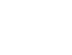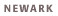Mathematics 640, 642

Degree Programs Offered: Master of Science, Doctor of Philosophy

Director of Graduate Program: Professor Charles A. Weibel, Hill Center for the Mathematical Sciences, Busch Campus (732/445-3864)

Eric Allender, Professor of Computer Science, FAS-NB; Ph.D., Georgia Institute of Technology
Complexity theory; parallel and probabilistic computation

Abbas Bahri, Professor of Mathematics, FAS-NB; Doctorat, École Normale Supérieure
Variational problems in nonlinear analysis and geometry

Tadeusz Balaban, Professor of Mathematics, FAS-NB; Ph.D., Warsaw
Mathematical physics

José Barros-Neto, Professor Emeritus of Mathematics, FAS-NB; Ph.D., Săo Paulo
Functional analysis; partial differential equations

R. Michael Beals, Professor of Mathematics, FAS-NB; Ph.D., Princeton
Harmonic analysis; Fourier integral operators; partial differential equations

József Beck, Harold H. Martin Professor of Mathematics, FAS-NB; Ph.D., Hungarian Academy of Sciences
Combinatorics, combinatorial games, number theory

Adi Ben-Israel, Professor of Management, SB-NB; Ph.D., Northwestern
Matrix theory, numerical linear algebra, convexity and optimization operations research, mathematical economics

Edward Boylan, Associate Professor of Mathematics, FAS-N; Ph.D., Princeton
Probability and computer science

Haim Brezis, Distinguished Visiting Professor of Mathematics, FAS-NB; Doctorat, Paris Nonlinear functional analysis; partial differential equations

Felix E. Browder, Professor of Mathematics, FAS-NB; Ph.D., Princeton
Functional analysis and partial differential equations

Richard T. Bumby, Professor of Mathematics, FAS-NB; Ph.D., Princeton
Number theory

Terence Butler, Professor of Mathematics, FAS-NB; Ph.D., Indiana
Differential equations

Lisa Carbone, Associate Professor of Mathematics, FAS-NB; Ph.D., Columbia Group action on trees

Sagun Chanillo, Professor of Mathematics, FAS-NB; Ph.D., Purdue
Classical analysis; partial differential equations

Gregory L. Cherlin, Professor of Mathematics, FAS-NB; Ph.D., Yale
Logic; model theory; model theoretic algebra

Vasek Chvátal, Professor of Computer Science, FAS-NB; Ph.D., Waterloo
Combinatorial optimization; combinatorics

Amy Cohen, Professor of Mathematics, UC-NB; FAS-NB; Ph.D., California (Berkeley)
Partial differential equations; inverse scattering; Korteweg-de Vries equation

Richard M. Cohn, Professor Emeritus of Mathematics, FAS-NB; Ph.D., Columbia
Differential algebra

Bernard Coleman, J. Willard Gibbs Professor of Thermomechanics, SE; Ph.D., Yale
Continuum mechanics, analysis

Jane Cronin, Professor Emerita of Mathematics, FAS-NB; Ph.D., Michigan
Qualitative theory of ordinary differential equations; biomathematics

Carl C. Faith, Professor Emeritus of Mathematics, FAS-NB; Ph.D., Purdue
Theory of rings and modules

Richard S. Falk, Chair of Department and Professor of Mathematics, FAS-NB; Ph.D., Cornell
Numerical analysis; partial differential equations

Paul Feehan, Associate Professor of Mathematics, FAS-NB; Ph.D., Columbia Partial differential equations; gauge theory and applications to four-manifolds; non-abelian monopoles

Steven Ferry, Joshua Barlaz Professor of Mathematics, FAS-NB; Ph.D., Michigan
Geometric topology; differential topology; differential geometry

Michael L. Fredman, Professor of Computer Science, FAS-NB; Ph.D., Stanford
Data structures and algorithms; computational complexity

Siqi Fu, Associate Professor of Mathematical Sciences, FAS-C; Ph.D., Washington Several complex variables

Giovanni Gallavotti, Distinguished Visiting Professor of Mathematics, FAS-NB; Ph.D., Florence
Statistical mechanics; quantum field theory; dynamical systems

Israel M. Gelfand, Professor of Mathematics, FAS-NB; Ph.D., Moscow State
Mathematics; artificial intelligence; neuroanatomy; cell biology

Jane P. Gilman, Professor of Mathematics, FAS-N; Ph.D., Columbia
Riemann surfaces; Fuchsian groups; Teichmuller theory; geometric topology; combinatorial group theory

Simon G. Gindikin, Professor of Mathematics, FAS-NB; Ph.D., Mathematics Institute (Leningrad)
Theory of representations; integral geometry; several complex variables; mathematical physics

Gerald A. Goldin, University Director, Science and Mathematics Partnerships, and Professor of Mathematics and Physics Education, GSE; Ph.D., Princeton Lie theory; mathematical physics; secondary education

Sheldon Goldstein, Professor of Mathematics, FAS-NB; Ph.D., Yeshiva
Statistical mechanics; probability theory

Roe Goodman, Professor of Mathematics, FAS-NB; Ph.D., Massachusetts
Institute of Technology Representation theory and analysis on Lie groups

Stephen A. Greenfield, Professor of Mathematics, FAS-NB; Ph.D., Brandeis
Linear partial differential equations; several complex variables

Richard F. Gundy, Professor of Statistics, FAS-NB; Ph.D., Chicago
Wavelets and time-frequency analysis; probability theory

András Hajnal, Professor Emeritus of Mathematics, FAS-NB; Ph.D., Bolyai Institute (Szeged) Combinatorics; mathematical logic; set theory

Peter Hammer, Professor of Mathematics, FAS-NB, and Director of the Rutgers Center for Operations Research; Ph.D., Bucharest Boolean methods in operations research; integer programming; applications of discrete mathematics; graph theory

Zheng-Chao Han, Associate Professor of Mathematics, FAS-NB; Ph.D., Courant Institute (New York)
Nonlinear analysis; partial differential equations

William Hoyt, Associate Professor Emeritus of Mathematics, FAS-NB; Ph.D., Chicago
Algebraic geometry; elliptic surfaces; modular forms

Xiaojun Huang, Professor of Mathematics, FAS-NB; Ph.D., Washington (St. Louis)
Several complex variables

Yi-Zhi Huang, Associate Professor of Mathematics, FAS-NB; Ph.D., Rutgers
Conformal field theory; mathematics underlying string theory

Henryk Iwaniec, State of New Jersey Professor of Mathematics, FAS-NB; Ph.D., Warsaw
Analytic number theory

Howard J. Jacobowitz, Professor of Mathematics, FAS-C; Ph.D., New York
Differential geometry

Jeffry N. Kahn, Professor of Mathematics, FAS-NB; Ph.D., Ohio State
Combinatorics and related fields

Johannes H.B. Kemperman, Professor Emeritus of Statistics, FAS-NB; Ph.D., Amsterdam
Probability and statistics

Michael Kiessling, Associate Professor of Mathematics, FAS-NB; Ph.D., Ruhr (Bochum)
Statistical mechanics

Friedrich Knop, Professor of Mathematics, FAS-NB; Ph.D., Basel (Switzerland)
Algebraic geometry; representation theory

János Komlós, Professor of Mathematics, FAS-NB; Ph.D., Eötvös
Combinatorics; probability; theoretical computer science

Maxim Kontsevich, Distinguished Visiting Professor of Mathematics, FAS-NB; Ph.D., Bonn
String theory and quantum field theory; quantum cohomology

Antoni A. Kosinski, Professor Emeritus of Mathematics, FAS-NB; Ph.D., Warsaw
Differential topology

Martin Kruskal, David Hilbert Professor of Mathematics, FAS-NB; Ph.D., New York
Soliton equations; asymptotic analysis; surreal numbers

Antti Kupiainen, Distinguished Visiting Professor of Mathematics, FAS-NB; Ph.D., Princeton
Mathematical physics; quantum field theory; statistical mechanics

Peter S. Landweber, Professor of Mathematics, FAS-NB; Ph.D., Harvard
Algebraic topology; bordism theory; generalized homology theory

Solomon Leader, Professor Emeritus of Mathematics, FAS-NB; Ph.D., Princeton
Analysis; abstract spaces

Joel L. Lebowitz, George William Hill Professor of Mathematics and Director of the Center for Mathematical Sciences Research, FAS-NB; Ph.D., Syracuse
Mathematical physics; statistical mechanics

James I. Lepowsky, Professor of Mathematics, FAS-NB; Ph.D., Massachusetts Institute of Technology
Representational theory; vertex operator algebras; mathematics underlying string theory

Norman Levitt, Professor of Mathematics, FAS-NB; Ph.D., Princeton
Differential and geometric topology; surgery theory; structural theory

Yanyan Li, Professor of Mathematics, FAS-NB; Ph.D., New York
Nonlinear analysis; partial differential equations

Feng Luo, Professor of Mathematics, FAS-NB; Ph.D., California (San Diego)
Topology and geometry

Richard N. Lyons, Professor of Mathematics, FAS-NB; Ph.D., Chicago
Simple groups

Diane Maclagan, Assistant Professor of Mathematics, FAS-NB; Ph.D., California (Berkeley) Commutative algebra and algebraic geometry

Stephen D. Miller, Associate Professor of Mathematics, FAS-NB; Ph.D., Princeton Automorphic forms; L-functions

Benjamin Muckenhoupt, Professor Emeritus of Mathematics, FAS-NB; Ph.D., Chicago
Harmonic analysis; orthogonal expansions; weighted norm inequalities

Roger Nussbaum, Professor of Mathematics, FAS-NB; Ph.D., Chicago
Nonlinear functional analysis

Daniel L. Ocone, Professor of Mathematics, FAS-NB; Ph.D., Massachusetts Institute of Technology
Stochastic processes; stochastic control; filtering

Michael E. O'Nan, Professor of Mathematics, FAS-NB; Ph.D., Princeton
Permutation groups; simple groups

Barbara L. Osofsky, Professor Emeritus of Mathematics, FAS-NB; Ph.D., Rutgers
Ring theory; homological algebra

Ted Petrie, Professor of Mathematics, FAS-NB; Ph.D., Princeton
Algebraic and differential topology; transformation groups; equivariant surgery; financial mathematics

Andras Prekopa, Professor of Operations Research and Statistics, FAS-NB; Ph.D., Budapest
Optimization of stochastic systems

John D. Randall, Associate Professor of Mathematics, FAS-N; Ph.D., Warwick
Topology; algebraic geometry

Vladimir Retakh, Associate Professor of Mathematics, FAS-NB; Ph.D., Moscow
Pedagogical Institute Noncommutative algebra and combinatorics; special functions and differential equations; mathematics education

Fred S. Roberts, Professor of Mathematics, FAS-NB, and Director of DIMACS; Ph.D., Stanford
Discrete mathematical models; graph theory; decision making; measurement theory

Xiaochun Rong, Associate Professor of Mathematics, FAS-NB; Ph.D., SUNY (Stony Brook)
Riemannian geometry

Joseph Rosenstein, Professor of Mathematics, FAS-NB, and Associate Director of Education, DIMACS; Ph.D., Cornell
Logic; linear orderings; recursive model theory

David Ruelle, Distinguished Visiting Professor of Mathematics, FAS-NB; Ph.D., Brussels
Statistical mechanics; dynamical systems

Siddhartha Sahi, Professor of Mathematics, FAS-NB; Ph.D., Yale
Representations of reductive groups

Michael Saks, Professor of Mathematics, FAS-NB; Ph.D., Massachusetts
Institute of Technology Combinatorial optimization and algorithms; extremal set theory; partially ordered sets

Vladimir Scheffer, Professor of Mathematics, FAS-NB; Ph.D., Princeton
Geometric measure theory; partial differential equations

David Shanno, Professor of Management, SB-NB; Ph.D., Carnegie Mellon
Mathematical programming, numerical analysis

Saharon Shelah, Distinguished Visiting Professor of Mathematics, FAS-NB; Ph.D., Hebrew (Jerusalem)
Logic; model theory

Diana F. Shelstad, Professor of Mathematics, FAS-N; Ph.D., Yale
Harmonic analysis on algebraic groups related to number theory and geometry

Lawrence Shepp, Professor of Statistics, FAS-NB; Ph.D., Princeton
Pure and applied probability; tomography; mathematics of finance

Charles C. Sims, Professor of Mathematics, FAS-NB; Ph.D., Harvard
Computational group theory and algebraic algorithms

Avraham Soffer, Professor of Mathematics, FAS-NB; Ph.D., Tel Aviv
Theory of partial differential evolution equations; Schrödinger operators and scattering theory; general mathematical physics

Eduardo D. Sontag, Professor of Mathematics, FAS-NB; Ph.D., Florida
System and control theory

Eugene R. Speer, Professor of Mathematics, FAS-NB; Ph.D., Princeton
Mathematical physics

Hector J. Sussmann, Professor of Mathematics, FAS-NB; Ph.D., New York
System and control theory

Endre Szemerédi, State of New Jersey Professor of Computer Science, FAS-NB; Ph.D., Budapest
Theoretical computer science; number theory; combinatorics

Earl J. Taft, Professor of Mathematics, FAS-NB; Ph.D., Yale
Hopf algebras

Nonlinear hyperbolic partial differential equations

Jean E. Taylor, Professor Emerita of Mathematics, FAS-NB; Ph.D., Princeton
Geometric measure theory

Simon Thomas, Professor of Mathematics, FAS-NB; Ph.D., London
Model theory; infinite groups

Myles Tierney, Professor Emeritus of Mathematics, FAS-NB; Ph.D., Columbia
Topos theory

Gabor Toth, Professor of Mathematics, FAS-C; Ph.D., Eötvös Loránd
Differential geometry

J. François Tręves, Robert Adrain Professor Emeritus of Mathematics, FAS-NB; Ph.D., Sorbonne
Functional analysis; linear partial differential equations; several complex variables

Jerrold B. Tunnell, Associate Professor of Mathematics, FAS-NB; Ph.D., Harvard
Number theory; automorphic forms

Wolmer V. Vasconcelos, Professor of Mathematics, FAS-NB; Ph.D., Chicago
Commutative algebra; computational algebra

Michael Vogelius, Board of Governors Professor of Mathematics, FAS-NB; Ph.D., Maryland
Numerical analysis; partial differential equations

Charles A. Weibel, Professor of Mathematics, FAS-NB; Ph.D., Chicago
Algebraic K-theory; homological algebra; algebraic topology; category theory

Richard L. Wheeden, Professor of Mathematics, FAS-NB; Ph.D., Chicago
Harmonic analysis; harmonic functions; weighted norm inequalities

Robert L. Wilson, Professor of Mathematics, FAS-NB; Ph.D., Yale
Lie algebras

Christopher T. Woodward, Associate Professor of Mathematics, FAS-NB; Ph.D., Massachusetts Institute of Technology
Symplectic geometry; geometric quantization

Norman Zabusky, State of New Jersey Professor of Computational Fluid
Dynamics, SE; Ph.D., California Institute of Technology Vortex dynamics in two and three dimensions

Doron Zeilberger, Board of Governors Professor of Mathematics, FAS-NB; Ph.D., Weizmann Institute Algebraic and enumerative combinatorics; experimental mathematics

Hyman J. Zimmerberg, Professor Emeritus of Mathematics, FAS-NB; Ph.D., Chicago
Boundary value problems

Associate Members of the Graduate Faculty

Martin Farach-Colton, Associate Professor of Computer Science, FAS-NB; Ph.D., Maryland Computational molecular biology; sequential and partial algorithms

William F. Keigher, Associate Professor of Mathematics, FAS-N; Ph.D., Illinois
Differential and commutative algebra, category theory; algebraic geometry

Haisheng Li, Assistant Professor of Mathematics, FAS-C; Ph.D., Rutgers
Vertex operator algebras; mathematics related to string theory

Jacob Sturm, Associate Professor of Mathematics, FAS-N; Ph.D., Princeton
Number theory, automorphic forms